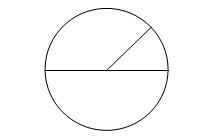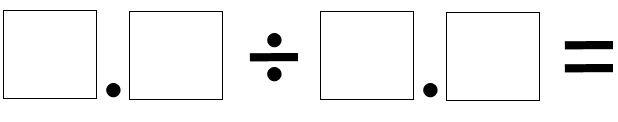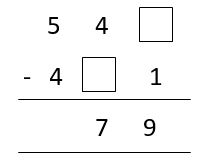# Area & Perimeter of Circles and Solving Number Puzzles

Area & Perimeter of Circles

While the knowledge of formulae is not expected at year six, or at least not as of now as I write this, there is every chance that an independent school will throw it in as a way of seeing how the better pupils deal with a complex question.

Firstly, let's name the elements of a circle that will get mentioned.

The line going from one side to the other is the diameter. The line from the edge to the middle is the radius. As the outside of the circle is always the same distance from the centre, the diameter is double the length of the radius.PERIMETER: The basic idea is to multiply the diameter of the circle by 3.14, a number known as pi, or π. The precise value of pi is endless and sometimes your child will be asked to assume it is 3.14 or even just 3.

The formula for the perimeter of the circle is:      π x d     or     2 x π x r (as diameter is the same as two lots of the radius)

If we use 3.14 to represent π then a circle of radius 4m will have a perimeter of:

2 x 3.14 x 4 = 25.12 metres.

AREA: The area is always shown in square units and is calculated by multiplying the radius by itself and π. If you know only the diameter, halve it to get the radius and then use the formula:

π x r x r     or     π r 2

Assuming π to be 3.14, a circle with radius 4m will have an area of:

3.14 x 4 x 4 = 50.24 m2

Solving Number Puzzles

There are many different questions that examiners can dream up but a common number puzzle that appears in SATs is one where a series of numbers is provided and a child has to arrange them to make sense as a sum.

For instance: Use the numbers 5, 7, 8 and 2 in any order to fill the boxes below. What order should they be put in to create the highest answer?Children may be expected to use trial and error to formulate an answer but there are some shortcuts.

When presented with a division sum, always divide by the lowest number possible to give the highest answer. The lowest number available would be 2.5. The highest number that could be created is 8.7 so the sum which gives the highest answer has to be 8.7 ÷ 2.5. Note that we have not worked out any sums at all and only need to have a cursory glance to check that our answer isn't silly.

Another puzzle might involve finding which numbers are missing from a sum, thus:The idea of trial and error on this one is redundant as there are mathematical shortcuts here.

Firstly, the “units” column is critical. Something take away one equals nine. This must be ten, so the number in the top box must be zero. Next, as we now have '541 - something = 79' we can simply take 79 from 541 to give the answer 461.

There are often shortcuts but, should all else fail and a child has no way in to the question, trial and error gives a starting point.

We use cookies to make your experience of our website better.

To comply with the new e-Privacy directive, we need to ask for your consent -